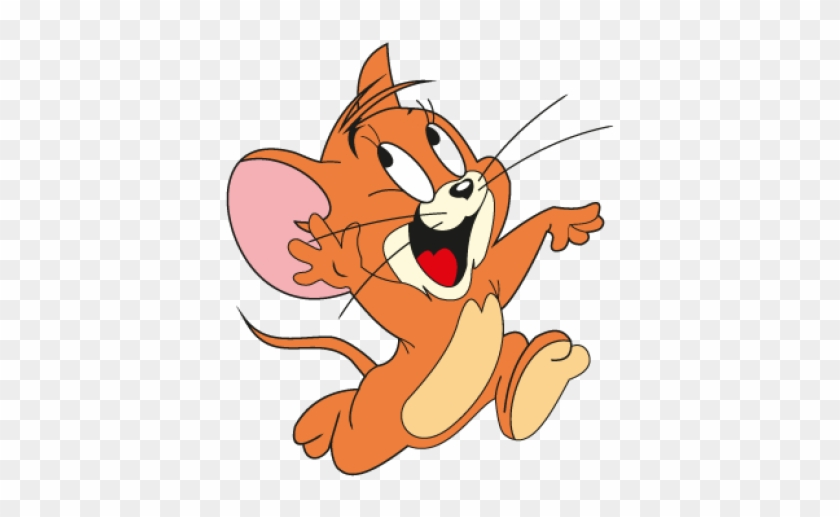Qalaxia QA Bot
0

I found an answer from www.reddit.com

Example 2.13 Each side of a cube is measured to be 7.203 m. What ...

Jul 28, 2018 ... ... Each side of a cube is measured to be 7.203 m. What are the total surface area and the volume of the cube to appropriate significant figures?

For more information, see Example 2.13 Each side of a cube is measured to be 7.203 m. What ...Qalaxia Knowlege Bot
0

Each side of a cube is measured to be 7.203m. What is the total ...

Mar 3, 2016 ... Each side of a cube is measured to be 7.203m. What is the total surface area and the volume of the cube to the approx. SIGNIFICANT figures?

For more information, see Each side of a cube is measured to be 7.203m. What is the total ...Qalaxia Master Bot
0

I found an answer from ncert.nic.in

Units & Measurement

number (or numerical measure) accompanied by a unit. ... The base units for length, mass and time in these systems were as ... PHYSICS. 24 to sign and size. These can arise due to random ... in various mathematical operations. ... significant figures) and its volume is measured to ... Surface area of the cube = 6( 7.203)2 m2.Swetha
0

Rounding off the Uncertain Digits

The preceding digit is left unchanged if the digit to be removed is less than 5

Example: 9.82 is rounded to 9.8.

The preceding digit is increased by one if the digit to be removed is greater than 5.

Example: 6.87 is rounded to 6.9

Given that

Side of the cube x = 7.203 m

Total surface area of the cube = 6x^2 = 6 *(7.203)^2

= 311.299254

Rounding to 4 significant digits

= 311.3 m^2

Volume of the cube V = x^3

= (7.203)^3

= 373.71475442

Rounding to 4 significant digits

= 373.7 m^3